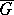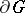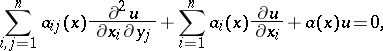# Harnack theorem

(diff) ← Older revision | Latest revision (diff) | Newer revision → (diff)
Harnack's first theorem: If a sequence of functions which are harmonic in a bounded domainand continuous onconverges uniformly on the boundary, then it also converges uniformly into a harmonic function. This theorem can be generalized to solutions of an elliptic equation,(*)
which has a unique solution of the Dirichlet problem for any continuous boundary function . If the sequence of solutions of equation (*) converges uniformly on, then it also converges uniformly into a solution of equation (*).
Harnack's second theorem, the Harnack principle: If a monotone sequence of harmonic functions in a bounded domainconverges at some point in, then it converges at all points ofto a harmonic function, and this convergence is uniform on any closed subdomain of. Harnack's second theorem can be generalized to monotone sequences of solutions of the elliptic equation .